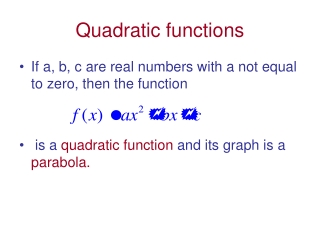DownloadDownload PresentationTélécharger la présentation- - - - - - - - - - - - - - - - - - - - - - - - - - - E N D - - - - - - - - - - - - - - - - - - - - - - - - - - -
Presentation Transcript

1. Quadratic functions • If a, b, c are real numbers with a not equal to zero, then the function • is a quadratic functionand its graph is a parabola.

2. Vertex form of the quadratic function • It is convenient to convert the general form of a quadratic equation • to what is known as the vertex form.

3. Completing the square to find the vertex of a quadratic function • The example below illustrates the procedure: Consider Complete the square to find the vertex:

4. Completing the square, continued • The vertex is (1 , 2) • The quadratic function opens down since the coefficient of the x squared term is negative (-3) .

5. Intercepts of a quadratic function • Find the x-intercepts of. • Set f(x) = 0 • Use the quadratic formula: X =

6. Intercepts of a quadratic function • X= Find the y-intercept : Let x = 0 and solve for y: We have (0, -1)

7. Generalization • Summary: • where a is not equal to zero. Graph of f is a parabola: if a > 0, the graph opens upward if a < 0 , the graph opens downward.

8. Generalization, continued • Vertex is (h , k) • Line or axis of symmetry: x = h • f(h) = k is the minimum if a > 0, otherwise, f(h) = k is the maximum • Domain : set of all real numbers • Range: if a < 0. If a > 0, the range is

9. Application of Quadratic Functions • A Macon Georgia peach orchard farmer now has 20 trees per acre. Each tree produces, on the average, 300 peaches. For each additional tree that the farmer plants, the number of peaches per tree is reduced by 10. How many more trees should the farmer plant to achieve the maximum yield of peaches? What is the maximum yield?

10. Solution • Solution: Yield= number of peaches per tree x number of trees • Yield = 300 x 20 = 6000 ( currently) • Plant one more tree: Yield = ( 300 – 1(10)) * ( 20 + 1) = 290 x 21 = 6090 peaches. • Plant two more trees: • Yield = ( 300 – 2(10)* ( 20 + 2) = 280 x 22 = 6160

11. Solution, continued • Let x represent the number of additional trees. Then Yield =( 300 – 10x) (20 + x)= •  Y(x)= • To find the maximum yield, note that the Y(x) function is a quadratic function opening downward. Hence, the vertex of the function will be the maximum value of the yield.

12. Solution, continued • Complete the square to find the vertex of the parabola: • Y(x) = • (we have to add 250 on the outside since we multiplied • –10 by 25 = -250. The equation is unchanged, then.

13. Solution,continued • Y(x)=   • Thus, the vertex of the quadratic function is ( 5 , 6250) . So the farmer should plant 5 additional trees and obtain a yield of 6250 peaches. We know this yield is the maximum of the quadratic function since the the value of a is -10. The function opens downward so the vertex must be the maximum.